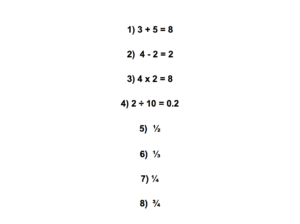## Since 2016

티앤잉글리쉬 예시문These numbers show how to express simple maths like addition, subtraction, multiplication and division. Number one is “Three plus five equals eight”. Number two is “Four minus two equals two”. Number three is “Four multiplied by two equals eight”. Number four is “Two divided by 10 equals zero point two”. Number five is a half. The next question is a third, number seven is a quarter and the last question is three quarters.

이 숫자들은 덧셈, 뺄셈, 곱셈, 나눗셈과 같은 간단한 수학을 어떻게 표현하는지에 대해 보여주네요. 1번은 3과 5를 더하면 8.  2번은 4에서 2를 빼면 2. 3번은 4곱하기 2는 8. 4번은 2나누기 10은 0.2. 5번은 2분의1, 6번은 3분의1, 7번은 4분의1, 마지막 번호는 4분의3을 보여줍니다.

Must remember expressions
• subtraction 뺄셈
•  multiplication 곱셈
• division 나눗셈

영어 일기 작성시 많이 틀리는 내용!

덧셈, 뺄셈, 곱셈, 나눗셈은 명사이며, 더하다 빼다 나누다 곱하다 라는 표현은 동사입니다. 아래 표를 확인해 주세요.

 Noun(명사) Verb(동사) Example(예시) Addition Plus Six plus three equals nine. Subtraction Minus Five minus two equals three. Multiplication Multiplied by / Times Eight multiplied by four equals thirty-two.Eight times four equals thirty-two. Division Divided by Twelve divided by three equals four.